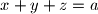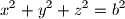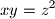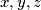### IMO Shortlist 1961 problem 1

Kvaliteta:
Avg: 0,0
Težina:
Avg: 4,0
Solve the system of equations:where$a$ and$b$ are constants. Give the conditions that$a$ and$b$ must satisfy so that$x,y,z$ are distinct positive numbers.
Izvor: Međunarodna matematička olimpijada, shortlist 1961